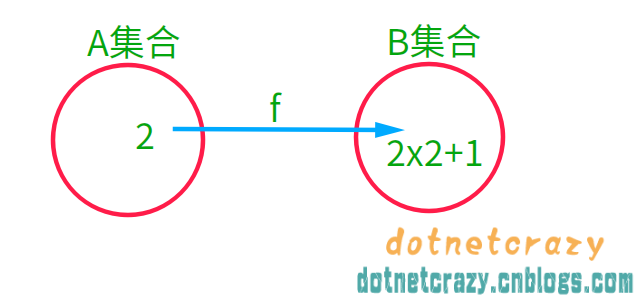# 码农眼中的数学之～数学基础

## 1.基础概念¶

1. 把2维世界转换成2维的世界
2. 把3维世界转换成2维的世界
3. 把2维世界转换成3维的世界

1维直线、2维平面（长宽）、3维空间（长宽高 | xyz轴）、4维时空（xyz轴+时间轴）

### 1.1.数的分类¶

• 自然数
• 负数

• 整数
• 正整数
• 0
• 负整数

1. 有理数（分数）
• 整数
• 正整数
• 0
• 负整数
• 非整数的有理数
2. 无理数

$$x = {-b \pm \sqrt{b^2-4ac} \over 2a}$$

In :
# 画个图看看曲线长什么样
import matplotlib.pyplot as plt

In :
# 生成x和y的值
x_list = list(range(-10, 11))
y_list = [x**2 + 3 for x in x_list] # 2**3 ==> 8 **是Python里面的幂运算符

print(x_list)
print(y_list)

# 画图
plt.plot(x_list, y_list)
# 显示图片
plt.show()


[-10, -9, -8, -7, -6, -5, -4, -3, -2, -1, 0, 1, 2, 3, 4, 5, 6, 7, 8, 9, 10]
[103, 84, 67, 52, 39, 28, 19, 12, 7, 4, 3, 4, 7, 12, 19, 28, 39, 52, 67, 84, 103]1. 实数（虚部b=0）
• 有理数
1. 整数
• 正整数：1、2、3
• 0
• 负整数：-1、-2、-3
2. 非整数的有理数（[正负]分数）
• [正负]有限小数：0.3 ==> (3/10)
• [正负]循环小数：0.3333... (1/3)
• 无理数
• 无限不循环小数：π、√3
2. 虚数（虚部b!=0）
• 纯虚数（虚部b!=0，且实部a=0）
• 非纯虚数

### 扩展：二次方程求解公式的推导¶

$\mathbf{ax^2+bx+c=0（a\neq0)}$

$\mathbf{x^2+\frac{b}{a}x+\frac{c}{a}=0}$

$\mathbf{x^2+\frac{b}{a}x=-\frac{c}{a}}$

$\mathbf{x^2+2*\frac{b}{2a}x+(\frac{b}{2a})^2=(\frac{b}{2a})^2-\frac{c}{a}}$

$\mathbf{(x+\frac{b}{2a})^2=(\frac{b}{2a})^2-\frac{c}{a}}$

$\mathbf{(x+\frac{b}{2a})^2=\frac{b^2}{4a^2}-\frac{4ac}{4a^2}=\frac{b^2-4ac}{4a^2}}$

$\mathbf{x+\frac{b}{2a}=\frac{ \pm \sqrt{b^2-4ac}}{2a}}$

$\mathbf{x=\frac{-b \pm \sqrt{b^2-4ac}}{2a}}$

$ax^2+bx+c=0（a\neq0)$

$x^2+\frac{b}{a}x+\frac{c}{a}=0$

$x^2+\frac{b}{a}x=-\frac{c}{a}$

$x^2+2*\frac{b}{2a}x+(\frac{b}{2a})^2=(\frac{b}{2a})^2-\frac{c}{a}$

$(x+\frac{b}{2a})^2=(\frac{b}{2a})^2-\frac{c}{a}$

$(x+\frac{b}{2a})^2=\frac{b^2}{4a^2}-\frac{4ac}{4a^2}=\frac{b^2-4ac}{4a^2}$

$x+\frac{b}{2a}=\frac{ \pm \sqrt{b^2-4ac}}{2a}$

$x=\frac{-b \pm \sqrt{b^2-4ac}}{2a}$

### 1.2.命题相关¶

1. P是Q的必要条件
2. Q是P的充分条件

1. P是Q的充分必要条件
2. Q是P的充分必要条件

### 1.3.集合系列¶

1. 确定性（集合中的元素必须是确定的）
2. 互异性（集合中的元素互不相同）eg：集合A={1，a}，则a不能等于1）
3. 无序性（集合中的元素没有先后之分）eg：集合{3,4,5}和{3,5,4}是同一个集合

1. X = {0, 2, 4, 6, 8}
2. X = {2n | n = 0, 1, 2, 3, 4}

In :
# Python3 Code
X = set([x for x in range(10) if x%2==0])

print(X)


{0, 2, 4, 6, 8}

In :
# 当x是X集合里面的元素时，可以表示为：x ∈ X
# eg:2 ∈ X
2 in X

Out:
True

eg：集合A：{1,2,3} 集合B：{1,2,3,4} ==> A ⊆ B

In :
A = set([1,2,3])
B = set([1,2,3,4])

print(A)
print(B)


{1, 2, 3}
{1, 2, 3, 4}

In :
# 子集（判断A是否是B的子集）
A.issubset(B)

Out:
True
In :
# 父集（B是否是A的父集）
B.issuperset(A)

Out:
True
In :
A = B

A.issubset(B)

Out:
True

In :
set1=set([1,2,5])
set2=set([2,4,6])

print(set1)
print(set2)


{1, 2, 5}
{2, 4, 6}

In :
# 交集 A∩B={x|x∈A,且x∈B}
set1 & set2

Out:
{2}
In :
# 并集 A∪B={x|x∈A,或x∈B}
set1 | set2

Out:
{1, 2, 4, 5, 6}
In :
# 差集 A-B={x∣x∈A,且x∉B}
set1 - set2

Out:
{1, 5}
In :
set3=set(list(range(10)))

print(set3)


{0, 1, 2, 3, 4, 5, 6, 7, 8, 9}

In :
# 【大前提】set2是set3的一个子集（set3包含于set2）
set2.issubset(set3)

Out:
True
In :
# 这时候求差集，就等于求补集
set3 - set2

Out:
{0, 1, 3, 5, 7, 8, 9}

### 1.4.映射系列（映射、像、定义域和值域、满单射、双射、逆映射、线性映射等）¶

#### 1.映射与像 ：¶

像这个说的还是有点抽象，举个简单的例子：

x=2 通过 f 形成的像是 2*2+1#### 2.值域和定义域：¶

x值组成的集合 {0、1、2...} 就叫做“映像f的定义域”。

1. g(f(x))=x
2. f(g(x))=x

#### 5.线性映射¶

1. $f(x_1)+f(x_2)=f(x_1+x_2)$
2. $cf(x_1)=f(cx_1)$

$f(x_1)+f(x_2)=x_1+x_2=f(x_1+x_2)$

$cf(x_1)=cx_1=f(cx_1)$

$f(x_1)+f(x_2)=x_1+x_2+2$

$f(x_1+x_2)=x_1+x_2+1$

$f(x_1)+f(x_2)\neq f(x_1+x_2)$

### 1.5.排列组合¶

1. 排列：从给定个数的元素中取出指定个数的元素进行排序
2. 组合：从给定个数的元素中仅仅取出指定个数的元素，不考虑排序

$\Large {C^r_n=\frac{n!}{r!(n-r)!}}$

$\Large {A^r_n=r!C^r_n=\frac{n!}{(n-r)!}}$

1.试问，他们从5个菜中选出3个不同的菜，有几种可能性？

ABC ABD ABE ACD ACE ADE
BCD BCE BDE
CDE

$\large {C^3_5=\frac{5!}{3!(5-3)!}=\frac{5×4×3×2×1}{3×2×1×2×1}=10}$

2.试问，选出的这3个菜有几种排放顺序

A ABC ACB
B BAC BCA
C CAB CBA

3.试问，从5个菜中选出3个不同的菜，并按顺序打包带走总共有多少种可能？

$\large {A^3_5=\frac{5!}{(5-3)!}=\frac{5×4×3×2×1}{2×1}=60}$

加法乘法两原理，贯穿始终的法则。与序无关是组合，要求有序是排列。

## 2.矩阵预告¶

posted @ 2018-07-12 08:13  鲲逸鹏  阅读(1995)  评论(7编辑  收藏